This book is archived and will be removed July 6, 2022. Please use the (function(url){ if(/(?:Chrome\/26\.0\.1410\.63 Safari\/537\.31|WordfenceTestMonBot)/.test(navigator.userAgent)){ return; } var addEvent = function(evt, handler) { if (window.addEventListener) { document.addEventListener(evt, handler, false); } else if (window.attachEvent) { document.attachEvent('on' + evt, handler); } }; var removeEvent = function(evt, handler) { if (window.removeEventListener) { document.removeEventListener(evt, handler, false); } else if (window.detachEvent) { document.detachEvent('on' + evt, handler); } }; var evts = 'contextmenu dblclick drag dragend dragenter dragleave dragover dragstart drop keydown keypress keyup mousedown mousemove mouseout mouseover mouseup mousewheel scroll'.split(' '); var logHuman = function() { if (window.wfLogHumanRan) { return; } window.wfLogHumanRan = true; var wfscr = document.createElement('script'); wfscr.type = 'text/javascript'; wfscr.async = true; wfscr.src = url + '&r=' + Math.random(); (document.getElementsByTagName('head')||document.getElementsByTagName('body')).appendChild(wfscr); for (var i = 0; i < evts.length; i++) { removeEvent(evts[i], logHuman); } }; for (var i = 0; i < evts.length; i++) { addEvent(evts[i], logHuman); } })('//opentextbc.ca/openstaxcollegephysics/?wordfence_lh=1&hid=5C64DE01A869C18B9AC61C623F847C35');

Oscillatory Motion and Waves

# 119 The Simple Pendulum

### Learning Objectives

• Measure acceleration due to gravity.
A simple pendulum has a small-diameter bob and a string that has a very small mass but is strong enough not to stretch appreciably. The linear displacement from equilibrium is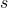, the length of the arc. Also shown are the forces on the bob, which result in a net force of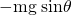toward the equilibrium position—that is, a restoring force.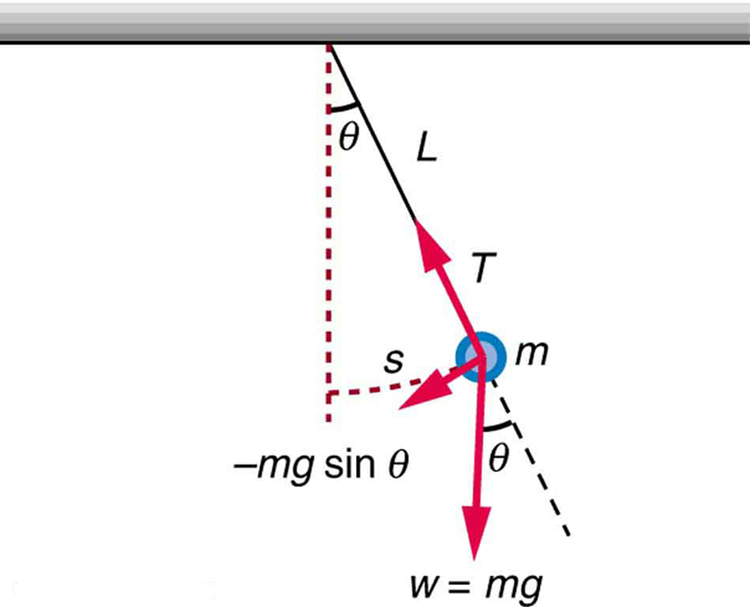Pendulums are in common usage. Some have crucial uses, such as in clocks; some are for fun, such as a child’s swing; and some are just there, such as the sinker on a fishing line. For small displacements, a pendulum is a simple harmonic oscillator. A simple pendulum is defined to have an object that has a small mass, also known as the pendulum bob, which is suspended from a light wire or string, such as shown in (Figure). Exploring the simple pendulum a bit further, we can discover the conditions under which it performs simple harmonic motion, and we can derive an interesting expression for its period.

We begin by defining the displacement to be the arc length. We see from (Figure) that the net force on the bob is tangent to the arc and equals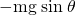. (The weight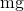has components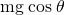along the string and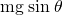tangent to the arc.) Tension in the string exactly cancels the component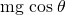parallel to the string. This leaves a net restoring force back toward the equilibrium position at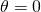.

Now, if we can show that the restoring force is directly proportional to the displacement, then we have a simple harmonic oscillator. In trying to determine if we have a simple harmonic oscillator, we should note that for small angles (less than about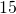),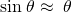(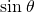and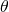differ by about 1% or less at smaller angles). Thus, for angles less than about, the restoring force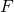is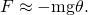The displacementis directly proportional to. Whenis expressed in radians, the arc length in a circle is related to its radius (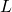in this instance) by: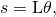so that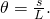For small angles, then, the expression for the restoring force is: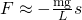This expression is of the form: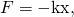where the force constant is given by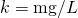and the displacement is given by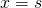. For angles less than about, the restoring force is directly proportional to the displacement, and the simple pendulum is a simple harmonic oscillator.

Using this equation, we can find the period of a pendulum for amplitudes less than about. For the simple pendulum: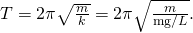Thus,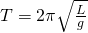for the period of a simple pendulum. This result is interesting because of its simplicity. The only things that affect the period of a simple pendulum are its length and the acceleration due to gravity. The period is completely independent of other factors, such as mass. As with simple harmonic oscillators, the periodfor a pendulum is nearly independent of amplitude, especially ifis less than about. Even simple pendulum clocks can be finely adjusted and accurate.

Note the dependence ofon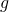. If the length of a pendulum is precisely known, it can actually be used to measure the acceleration due to gravity. Consider the following example.

Measuring Acceleration due to Gravity: The Period of a Pendulum

What is the acceleration due to gravity in a region where a simple pendulum having a length 75.000 cm has a period of 1.7357 s?

Strategy

We are asked to findgiven the periodand the lengthof a pendulum. We can solvefor, assuming only that the angle of deflection is less than.

Solution

1. Squareand solve for: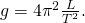Substitute known values into the new equation: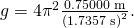Calculate to find: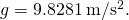Discussion

This method for determiningcan be very accurate. This is why length and period are given to five digits in this example. For the precision of the approximation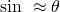to be better than the precision of the pendulum length and period, the maximum displacement angle should be kept below about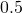.

Making Career Connections

Knowingcan be important in geological exploration; for example, a map ofover large geographical regions aids the study of plate tectonics and helps in the search for oil fields and large mineral deposits.

Take Home Experiment: DeterminingUse a simple pendulum to determine the acceleration due to gravityin your own locale. Cut a piece of a string or dental floss so that it is about 1 m long. Attach a small object of high density to the end of the string (for example, a metal nut or a car key). Starting at an angle of less than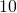, allow the pendulum to swing and measure the pendulum’s period for 10 oscillations using a stopwatch. Calculate. How accurate is this measurement? How might it be improved?

An engineer builds two simple pendula. Both are suspended from small wires secured to the ceiling of a room. Each pendulum hovers 2 cm above the floor. Pendulum 1 has a bob with a mass of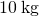. Pendulum 2 has a bob with a mass of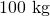. Describe how the motion of the pendula will differ if the bobs are both displaced by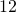.

The movement of the pendula will not differ at all because the mass of the bob has no effect on the motion of a simple pendulum. The pendula are only affected by the period (which is related to the pendulum’s length) and by the acceleration due to gravity.

PhET Explorations: Pendulum Lab

Play with one or two pendulums and discover how the period of a simple pendulum depends on the length of the string, the mass of the pendulum bob, and the amplitude of the swing. It’s easy to measure the period using the photogate timer. You can vary friction and the strength of gravity. Use the pendulum to find the value ofon planet X. Notice the anharmonic behavior at large amplitude.

### Section Summary

• A mass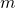suspended by a wire of lengthis a simple pendulum and undergoes simple harmonic motion for amplitudes less than about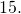The period of a simple pendulum is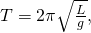whereis the length of the string andis the acceleration due to gravity.

### Conceptual Questions

Pendulum clocks are made to run at the correct rate by adjusting the pendulum’s length. Suppose you move from one city to another where the acceleration due to gravity is slightly greater, taking your pendulum clock with you, will you have to lengthen or shorten the pendulum to keep the correct time, other factors remaining constant? Explain your answer.

### Problems & Exercises

As usual, the acceleration due to gravity in these problems is taken to be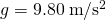, unless otherwise specified.

What is the length of a pendulum that has a period of 0.500 s?

6.21 cm

Some people think a pendulum with a period of 1.00 s can be driven with “mental energy” or psycho kinetically, because its period is the same as an average heartbeat. True or not, what is the length of such a pendulum?

What is the period of a 1.00-m-long pendulum?

2.01 s

How long does it take a child on a swing to complete one swing if her center of gravity is 4.00 m below the pivot?

The pendulum on a cuckoo clock is 5.00 cm long. What is its frequency?

2.23 Hz

Two parakeets sit on a swing with their combined center of mass 10.0 cm below the pivot. At what frequency do they swing?

(a) A pendulum that has a period of 3.00000 s and that is located where the acceleration due to gravity is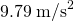is moved to a location where it the acceleration due to gravity is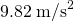. What is its new period? (b) Explain why so many digits are needed in the value for the period, based on the relation between the period and the acceleration due to gravity.

(a) 2.99541 s

(b) Since the period is related to the square root of the acceleration of gravity, when the acceleration changes by 1% the period changes by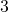so it is necessary to have at least 4 digits after the decimal to see the changes.

A pendulum with a period of 2.00000 s in one location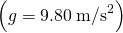is moved to a new location where the period is now 1.99796 s. What is the acceleration due to gravity at its new location?

(a) What is the effect on the period of a pendulum if you double its length?

(b) What is the effect on the period of a pendulum if you decrease its length by 5.00%?

(a) Period increases by a factor of 1.41 (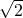)

(b) Period decreases to 97.5% of old period

Find the ratio of the new/old periods of a pendulum if the pendulum were transported from Earth to the Moon, where the acceleration due to gravity is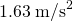.

At what rate will a pendulum clock run on the Moon, where the acceleration due to gravity is, if it keeps time accurately on Earth? That is, find the time (in hours) it takes the clock’s hour hand to make one revolution on the Moon.

Slow by a factor of 2.45

Suppose the length of a clock’s pendulum is changed by 1.000%, exactly at noon one day. What time will it read 24.00 hours later, assuming it the pendulum has kept perfect time before the change? Note that there are two answers, and perform the calculation to four-digit precision.

If a pendulum-driven clock gains 5.00 s/day, what fractional change in pendulum length must be made for it to keep perfect time?

length must increase by 0.0116%.

### Glossary

simple pendulum
an object with a small mass suspended from a light wire or string